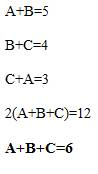# TIME AND WORK PART-2-LEARN SERIES

Hi Bankersdaily Aspirants,

Learning is a step by step process and you should gradually progress in that to ace the process. As you all know we have started a new Learn Series to help with your preparations for various exams like IBPS PO , IBPS RRB Scale I officer , IBPS RRB Office Assistant , IBPS Clerk , IBPS SO , SBI PO , SBI Clerk , SBI SO and many other exams in this type. Nevertheless this series will also contribute you all the tips and tricks to every miscellaneous sections and also all the important topics which are asked in the exams. If you haven’t looked at the Learn series , Please Click the below link to learn the new concepts.

Also we are coming daily with different topics and if you haven’t checked our latest article on the Problems based on Time and Work  Part 1,Please Click the link below

### INTRODUCTION TO TIME AND WORK:

There will be 1 or 2 Questions from this topic in all IBPS Exams.This topic is quite easy,Since Time and Work can be solved in Partial Method in our article we use LCM method to solve the problem in order to reduce the time consumption.There are 8 Basic types in Time and Work.Let us discuss each type with an example.

## #.4 TYPE 4:

### PROBLEMS BASED ON ALTERNATE DAYS:

In this type,we will be given individual Efficiency and the we are asked to find out the number of days when they work Alternatively.

1) A can complete the whole work in 10 days in the same time B can complete the work in 8 days.Then find in how many days they would complete the work if they work in alternate days?

Explanation

Given that,

A can complete the whole work in 10 days in the same time B can complete the work in 8 days

Total Work=LCM(10,8)=40

Efficiency of A=40/10=4 work/day

Efficiency of B=40/8=5 work/day

So,first day A will work and 2nd day B will work

So in 8 days they complete 36 works,Remaining 4 work is completed by A in the next day that is (9th

day)

Therefore,They will complete the task in 9 days

## #.5. TYPE 5:

### PROBLEMS BASED ON EFFICIENCY:

In this Type,we will be given any one’s individual efficiency and relation between the individual person and another person.(More Often they will indicate the relation between the two person as Twice,Thrice,..Sometimes with Percentage)

1) B can Complete the work in 10 days and A is twice as efficient as B.Find how long will A take to complete the work when he works alone?

Explanation

Given that,

B can Complete the work in 10 days

Total Work=LCM(2,10)=10 work

B’s Efficiency =10/10=1 work/day

Given that A is twice as efficient as B,

A=2(B)

A=2(1) work/day

So A can Complete the work in=10/2 = 5 days

## #.6. TYPE 6:

### PROBLEMS BASED ON FINDING INDIVIDUAL AND TOGETHER WORK FROM COMBINED WORK:

In this type ,we will be given the combined work and we are asked to find the individual work and the number of days when they work together.

1)A and B work together and complete the work in 12 days,B and C work together and complete the work in 15 days while C and A work together and complete the work in 20 days.Find in how many days they will complete the work if they work alone and if they work together?

Explanation

Given that,

A and B=12 days

B and C=15 days

C and A=20 days

Total Work=LCM(12,15,20)=60 work

Efficiency of A and B=60/12 = 5 work/day

Efficiency of B and C=60/15=4 work/day

Efficiency of C and A=60/20 = 3 work/dayBy Solving the Efficiency,

we get,

Efficiency of A=2 work/day

Efficiency of B=3 work/day

Efficiency of C=1 work/day

Efficiency of A+B+C=6 work/day

So,the Time Taken by A=60/2=30 days

Time Taken by B=60/3 =20 days

Time Taken by C=60/1=60 days

Time Taken by A+B+C=60/6=10 days

## You May Like to Read Other Articles:

HOW TO SOLVE SYLLOGISM USING VENN DIAGRAM METHOD?

HOW TO SOLVE COMBINATIONS-LEARN SERIES

SIMPLE INTEREST AND COMPOUND INTEREST